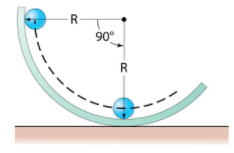# Problem: A ball of radius r rolls on the inside of a track of radius R(see the figure).Part AIf the ball starts from rest at the vertical edge of the track, what will be its speed when it reaches the lowest point of the track, rolling without slipping?Express your answer in terms of the variables R, r, and the constant g.

###### FREE Expert Solution

In this problem, we'll apply the conservation of energy equation:

$\overline{){{\mathbf{K}}}_{{\mathbf{0}}}{\mathbf{+}}{{\mathbf{U}}}_{{\mathbf{0}}}{\mathbf{+}}{{\mathbf{W}}}_{{\mathbf{nc}}}{\mathbf{=}}{{\mathbf{K}}}_{{\mathbf{f}}}{\mathbf{+}}{{\mathbf{U}}}_{{\mathbf{f}}}}$, where Wnc is the work done by non-conservative forces such as friction.

There are no non-conservative forces in our case.

So, Wnc = 0

Also, the ball starts from rest, so K0 = 0.

87% (38 ratings)###### Problem Details

A ball of radius r rolls on the inside of a track of radius R(see the figure).Part A

If the ball starts from rest at the vertical edge of the track, what will be its speed when it reaches the lowest point of the track, rolling without slipping?

Express your answer in terms of the variables R, r, and the constant g.